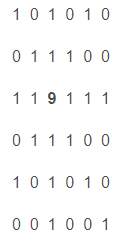用栈实现八皇后问题

国际象棋中皇后势力的范围是其所在位置的水平线、垂直线以及两条对角线。就像下面这样其中的  9 就表示皇后，其中的  1  表示皇后的势力范围。于是就产生了一个问题，在 n * n 的一个棋盘上，最多只能放置 n 个皇后使得他们不能互相攻击。解决这个问题的方式可以为先放置一个皇后在（0,0）处，之后在下一排下一位放置下一个皇后，不行的话再向后挪一位，然后再向下一排放置下一个皇后，但是当某一排皇后找不到合适位置时，就回到上一排，再挪动上一个皇后，一直到  n 个皇后放置完。这其中最主要的就是递归的调用以及栈的特性的使用。具体实现代码如下：

// ConsoleApplication1.cpp : 定义控制台应用程序的入口点。
//
#include "stdafx.h"
#include <iostream>
#include<cmath>
using namespace std;
#define StackSize 8 /*最多放8个皇后*/
int queen[StackSize][StackSize] = { 0 }; /*棋盘*/
int ans = 0;                           /*解数*/
int top = -1;                          /*栈顶指针*/
int datas;                  /*存储皇后位置*/
/*入栈*/
void Push(int x)                                          //入栈操作
{
top++;                                                           //栈顶指针上移
datas[top] = x;
}
/*出栈*/
void Pop()                                              //出栈操作
{
top--;                                                           //栈顶指针下移
}

void SeqStack() { top = -1; }                                              //构造函数，初始化空栈
/*放N皇后的递归函数*/
void PlaceQueen(int row)                                //在栈顶放置符合条件的值的操作,即摆放皇后
{
bool Judgement();
void SeqStack();                                    //关键点1：每一次摆放都会初始化空栈
void Output();
for (int col = 0; col<StackSize; col++)                              //穷尽0~7，即穷尽列
{
Push(col);
if (Judgement())                                             //判断摆放皇后的位置是否安全
{
if (row<StackSize - 1)                                     //若还没有放到第八个皇后，则进行下一个皇后的放置
PlaceQueen(row + 1);
else
{
ans++;                                               //解数加1
Output();                                            //打印成功的棋盘
}
}
Pop();                                                       //若不符合条件则出栈
}
}

/*关键点2：判断合法性*/
bool Judgement()
{
for (int i = 0; i<top; i++)                                           //依次检查前面各行的皇后位置
if (datas[top] == datas[i] || (abs(datas[top] - datas[i])) == (top - i))    //判断是否在同一列同一斜线
return false;                                            /*第一个条件，使每次入栈的数字不同层（行）*/
return true;                                                     /*top-i的值就是皇后之间的非法相对距离，值为0、1、2....，也就是同一条对角线或者斜对角线。data[top]-data[i]为2个皇后的实际的相对距离。*/
}

/*输出棋盘*/
void Output()                                          //将栈的数组形式打印成棋盘形式
{
cout << "NO." << ans << ":" << endl;
for (int i = 0; i<StackSize; i++)
{
for (int j = 0; j<datas[i]; j++)
cout << "- ";                                             //不放置处打印“-”
cout << "Q";                                                  //放置处打印“Q”
for (int j = StackSize - 1; j>datas[i]; j--)
cout << " -";
cout << endl;                                                 //换行
}
cout << endl;
}
int main()
{
PlaceQueen(0);                                        //从栈底开始赋值
cout << "the total number of solutions is:" << ans << endl;       //输出摆放方法的总数
system("pause");
return 0;
}